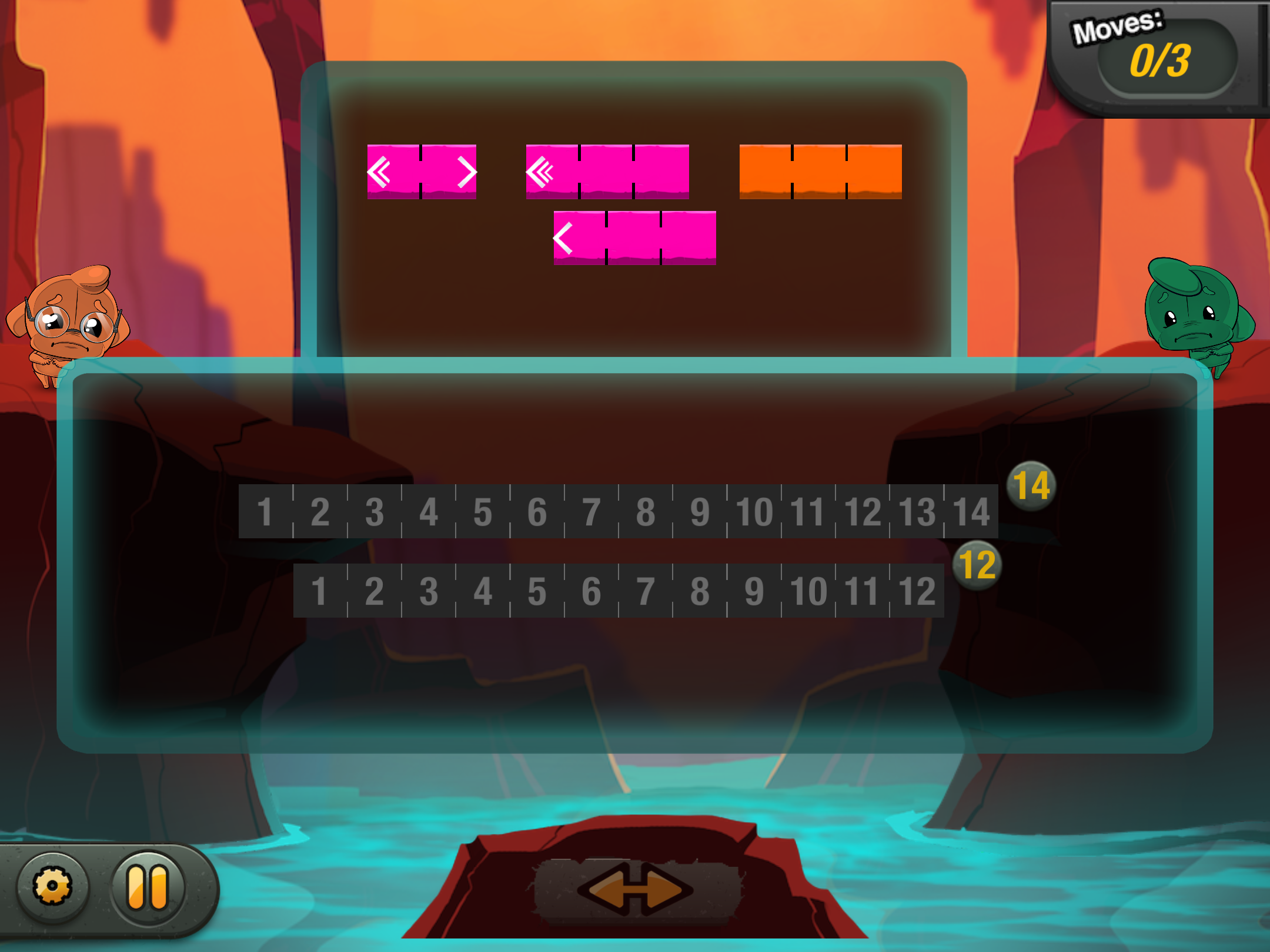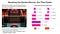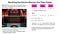# What’s the big idea behind the Tiles puzzle?A Tiles puzzle in the BrainQuake app. Drag some of the tiles into the trays so that, by one or more applications of the GROW command, both trays are exactly filled without any gaps or overlaps. The chevrons indicate the number of squares by which a tile will expand when you hit GROW. This puzzle requires reasoning about linear growth and breaks the Symbol Barrier for solving equations involving linear functions. Image by BrainQuake.

The first puzzle that greets you when you launch the new BrainQuake app is called Tiles. Puzzles involving the placement and movement of square or rectangular tiles on a board have a long history, starting with physical puzzles made of wood, cardboard, or plastic, and more recently digital versions. Many of them involve a combination of some (elementary) arithmetic and spatial reasoning, but they are primarily logical puzzles.

From a mechanistic perspective, BrainQuake’s Tiles puzzle was designed in a similar fashion. But it has an additional purpose: To familiarize players with the important mathematical concepts of linear functions, linear growth, and the solution of (linear) algebraic equations — while avoiding the more familiar approach of first teaching students how to write symbolic algebraic equations and coaching them in the procedures required to manipulate and solve them.

As I explained in the April 11 post, we refer to this approach as “breaking the symbol barrier,” in reference to the known linguistic problem (the symbol barrier) learners encounter when trying to make sense of new mathematical concepts and procedures. The tiles in the Tiles puzzle are non-algebraic representations of linear functions, as illustrated below.The tiles in the puzzle are representations of linear functions. Solving the puzzle involves reasoning about the growth pattern specified by the linear equations. To obtain a good score, players have to use as few GROW moves as possible, which requires careful selection of tiles from the palette and their placement in the trays. Image by BrainQuake.

The primary purpose is not to help the student learn how to “do algebra.” As I explained in the April 17 post, our games are designed to develop deep understanding of key mathematical concepts, mathematical thinking capacity (including algebraic thinking), and general problem-solving ability. The mathematical concepts on which each of our puzzles is based are simply vehicles to support learning of those higher-level capacities. (The earlier post provides links to independent, peer-reviewed university studies that show our approach works.)

A student who successfully works their way through the levels of the Tiles puzzle will not automatically find they can manipulate linear algebraic equations. What they will acquire is a deep, almost tactile understanding of linear growth and linear functions — one of the most important concepts in modern mathematics — and the ability to reason logically about the linear growth concept and solve problems involving linear growth.

Once a student has understood the key concepts, mastering the traditional symbolic language used to describe and solve problems involving linear growth is more readily achieved, just as people find it easier to learn to read music after they have first learned to play an instrument.

Note that I did not say mastering symbolic algebra is made easy by playing the Tiles game, any more than learning to play a guitar makes it easy to learn to read music. What playing BrainQuake does is provide meaning to, and understanding of, the symbolic representations when they are subsequently introduced (as occurs with music). We break the Symbol Barrier.

[BrainQuake has also designed and built digital tools to facilitate subsequent mastery of the symbolic language, which I will discuss in future posts to this blog, after that feature has been made available.]

Having, I hope, made it clear that the goal is not to develop the ability to do symbolic algebra, I will however leave you with an illustration that indicates how solving a Tiles puzzle does involve the conceptual logical steps of solving the corresponding algebraic equations.Solving a Tiles puzzles involves the logical reasoning that is required to solve the corresponding algebraic equations. Image by BrainQuake.

Because the Tiles puzzle simulates a physical device, the corresponding system of algebraic equations appears more complicated than is usually encountered in school algebra. That would be problematic if the puzzle were intended to teach students how to do algebra, as it is usually understood (namely as symbol manipulation). But as I indicated above, the goal is to develop algebraic thinking.

Incidentally, the first ever algebra textbook, written by the Persian mathematician Muhammad ibn Musa al-Khwarizmi some time between 813 and 833 CE (the name “algebra” comes from the Arabic term al-jabr that appeared in the book’s title), did not use abstract symbols. It was written entirely in prose. In modern terminology, it was a textbook on algebraic thinking. You don’t need symbols to “do algebra” any more than you need musical notation to play an instrument.

– Keith

Developing children’s true math proficiency

## More from BrainQuake

Developing children’s true math proficiency